# Bidirectional-LSTM based RNNs for text-to-speech synthesis (en)¶

Source code: https://github.com/r9y9/nnmnkwii_gallery

In this notebook, we will investigate bidirectional-LSTM based Recurrent Neural Networks (RNNs). You will learn how to iterate dataset in sequence-wise (i.e., utterance-wise) manner instead of frame-wise. Dataset preparation parts are almost same as the DNN text-to-speech synthesis notebook. If you have already read the DNN text-to-speech synthesis notebook, you can skip Data section.

Generated audio examples are attached at the bottom of the notebook. For simplicity, feature extraction steps will be performed with an external python script (200 lines). To run the notebook, in addition to nnmnkwii and its dependencies, you will need the following packages:

Please make sure that you have all dependneices if you are trying to run the notebook locally.

pip install pysptk pyworld librosa tqdm docopt


Part of code is adapted from Merlin. Speech analysis/synthesis is done by pysptk and pyworld. Librosa is used to visualize features. PyTorch is used to build DNN models.

The notebook requires wav files with aligned HTS-style full-context lablel files. You can download the necessary files by the following script.

:

! ./scripts/download_data.sh slt_arctic_full_data # no-op if have already downloaded

slt_arctic_full_data already downloaded

:

%pylab inline
rcParams["figure.figsize"] = (16,5)

from nnmnkwii.datasets import FileDataSource, FileSourceDataset
from nnmnkwii.preprocessing import trim_zeros_frames, remove_zeros_frames
from nnmnkwii.preprocessing import minmax, meanvar, minmax_scale, scale
from nnmnkwii import paramgen
from nnmnkwii.io import hts
from nnmnkwii.frontend import merlin as fe
from nnmnkwii.postfilters import merlin_post_filter

from os.path import join, expanduser, basename, splitext, basename, exists
import os
from glob import glob
import numpy as np
from scipy.io import wavfile
from sklearn.model_selection import train_test_split
import pyworld
import pysptk
import librosa
import librosa.display
import IPython
from IPython.display import Audio

Populating the interactive namespace from numpy and matplotlib


## Data¶

In this demo we construct datasets from pre-computed linguistic/duration/acoustic features because computing features from wav/label files on-demand are peformance heavy, particulary for acoustic features. See the following python script if you are interested in how we extract features.

:

DATA_ROOT = "./data/slt_arctic_full_data"
test_size = 0.112
random_state = 1234

:

! python ./scripts/prepare_features.py ./data/slt_arctic_full_data

Features for duration model training found, skipping feature extraction.
Features for acousic model training found, skipping feature extraction.


### Data specification¶

Almost same as Merlin’s slt_arctic demo. The only difference is that frequency warping paramter alpha is set to 0.41, instead of 0.58. As far as I know 0.41 is the best parameter approximating mel-frequency axis for 16kHz-sampled audio signals.

:

mgc_dim = 180
lf0_dim = 3
vuv_dim = 1
bap_dim = 3

duration_linguistic_dim = 416
acoustic_linguisic_dim = 425
duration_dim = 5
acoustic_dim = mgc_dim + lf0_dim + vuv_dim + bap_dim

fs = 16000
frame_period = 5
hop_length = 80
fftlen = 1024
alpha = 0.41

mgc_start_idx = 0
lf0_start_idx = 180
vuv_start_idx = 183
bap_start_idx = 184

windows = [
(0, 0, np.array([1.0])),
(1, 1, np.array([-0.5, 0.0, 0.5])),
(1, 1, np.array([1.0, -2.0, 1.0])),
]


### File data sources¶

We need to specify 1) where to find pre-computed features and 2) how to process them. In this case,

1. collect_files : Collects .bin files. External python script writes files in binary format. Also we split the files into train/test set.

2. collect_features : Just load from file by np.fromfile.

:

class BinaryFileSource(FileDataSource):
def __init__(self, data_root, dim, train):
self.data_root = data_root
self.dim = dim
self.train = train
def collect_files(self):
files = sorted(glob(join(self.data_root, "*.bin")))
files = files[:len(files)-5] # last 5 is real testset
train_files, test_files = train_test_split(files, test_size=test_size,
random_state=random_state)
if self.train:
return train_files
else:
return test_files
def collect_features(self, path):
return np.fromfile(path, dtype=np.float32).reshape(-1, self.dim)

:

X = {"duration":{}, "acoustic": {}}
Y = {"duration":{}, "acoustic": {}}
utt_lengths = {"duration":{}, "acoustic": {}}
for ty in ["duration", "acoustic"]:
for phase in ["train", "test"]:
train = phase == "train"
x_dim = duration_linguistic_dim if ty == "duration" else acoustic_linguisic_dim
y_dim = duration_dim if ty == "duration" else acoustic_dim
X[ty][phase] = FileSourceDataset(BinaryFileSource(join(DATA_ROOT, "X_{}".format(ty)),
dim=x_dim,
train=train))
Y[ty][phase] = FileSourceDataset(BinaryFileSource(join(DATA_ROOT, "Y_{}".format(ty)),
dim=y_dim,
train=train))
utt_lengths[ty][phase] = np.array([len(x) for x in X[ty][phase]], dtype=np.int)


Then we can construct datasets for duration and acoustic models. We wil have

• X: Input (duration, acoustic) datasets

• Y: Target (duration, acoustic) datasets

Note that dataset itself doesn’t keep features in memory. It loads features on-demand while iteration or indexing.

For mini-batch sequenceial training (which should be drastically faster than batch_size=1), later we will use PackedSequence in PyTorch. For this, re-create datasets with PaddedFileSourceDataset.

:

for ty in ["duration", "acoustic"]:
for phase in ["train", "test"]:
train = phase == "train"
x_dim = duration_linguistic_dim if ty == "duration" else acoustic_linguisic_dim
y_dim = duration_dim if ty == "duration" else acoustic_dim
dim=x_dim,
train=train),
np.max(utt_lengths[ty][phase]))
dim=y_dim,
train=train),
np.max(utt_lengths[ty][phase]))


### Utterance lengths¶

Let’s see utterance lengths histrogram.

:

print("Total number of utterances:", len(utt_lengths["duration"]["train"]))
print("Total number of frames:", np.sum(utt_lengths["duration"]["train"]))
hist(utt_lengths["duration"]["train"], bins=64);

Total number of utterances: 1000
Total number of frames: 32006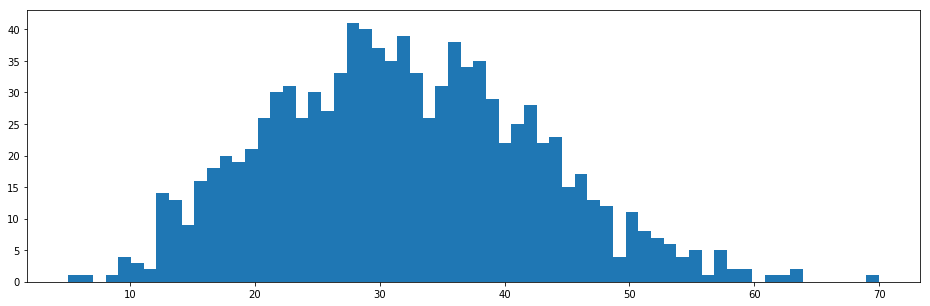:

print("Total number of utterances:", len(utt_lengths["acoustic"]["train"]))
print("Total number of frames:", np.sum(utt_lengths["acoustic"]["train"]))
hist(utt_lengths["acoustic"]["train"], bins=64);

Total number of utterances: 1000
Total number of frames: 534363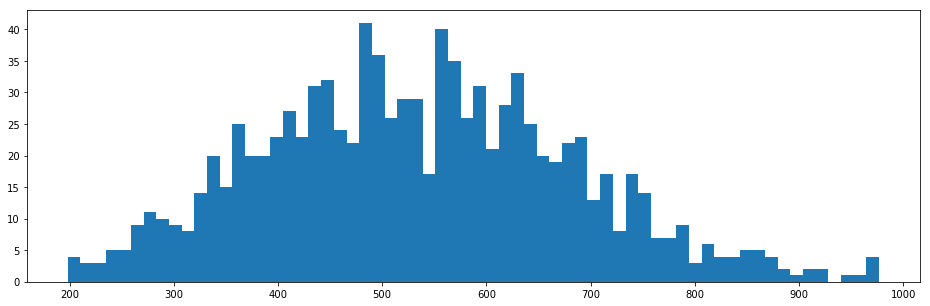### How data look like?¶

Pick an utterance from training data and visualize its features.

:

def vis_utterance(X, Y, lengths, idx):
"""Visualize the following features:

1. Linguistic features
2. Spectrogram
3. F0
4. Aperiodicity
"""
x = X[idx][:lengths[idx]]
y = Y[idx][:lengths[idx]]

figure(figsize=(16,20))
subplot(4,1,1)
# haha, better than text?
librosa.display.specshow(x.T, sr=fs, hop_length=hop_length, x_axis="time")

subplot(4,1,2)
logsp = np.log(pysptk.mc2sp(y[:,mgc_start_idx:mgc_dim//len(windows)], alpha=alpha, fftlen=fftlen))
librosa.display.specshow(logsp.T, sr=fs, hop_length=hop_length, x_axis="time", y_axis="linear")

subplot(4,1,3)
lf0 = y[:,mgc_start_idx]
vuv = y[:,vuv_start_idx]
plot(lf0, linewidth=2, label="Continuous log-f0")
plot(vuv, linewidth=2, label="Voiced/unvoiced flag")
legend(prop={"size": 14}, loc="upper right")

subplot(4,1,4)
bap = y[:,bap_start_idx:bap_start_idx+bap_dim//len(windows)]
bap = np.ascontiguousarray(bap).astype(np.float64)
aperiodicity = pyworld.decode_aperiodicity(bap, fs, fftlen)
librosa.display.specshow(aperiodicity.T, sr=fs, hop_length=hop_length, x_axis="time", y_axis="linear")
# colorbar()

:

idx = 0
vis_utterance(X["acoustic"]["train"], Y["acoustic"]["train"], utt_lengths["acoustic"]["train"], idx)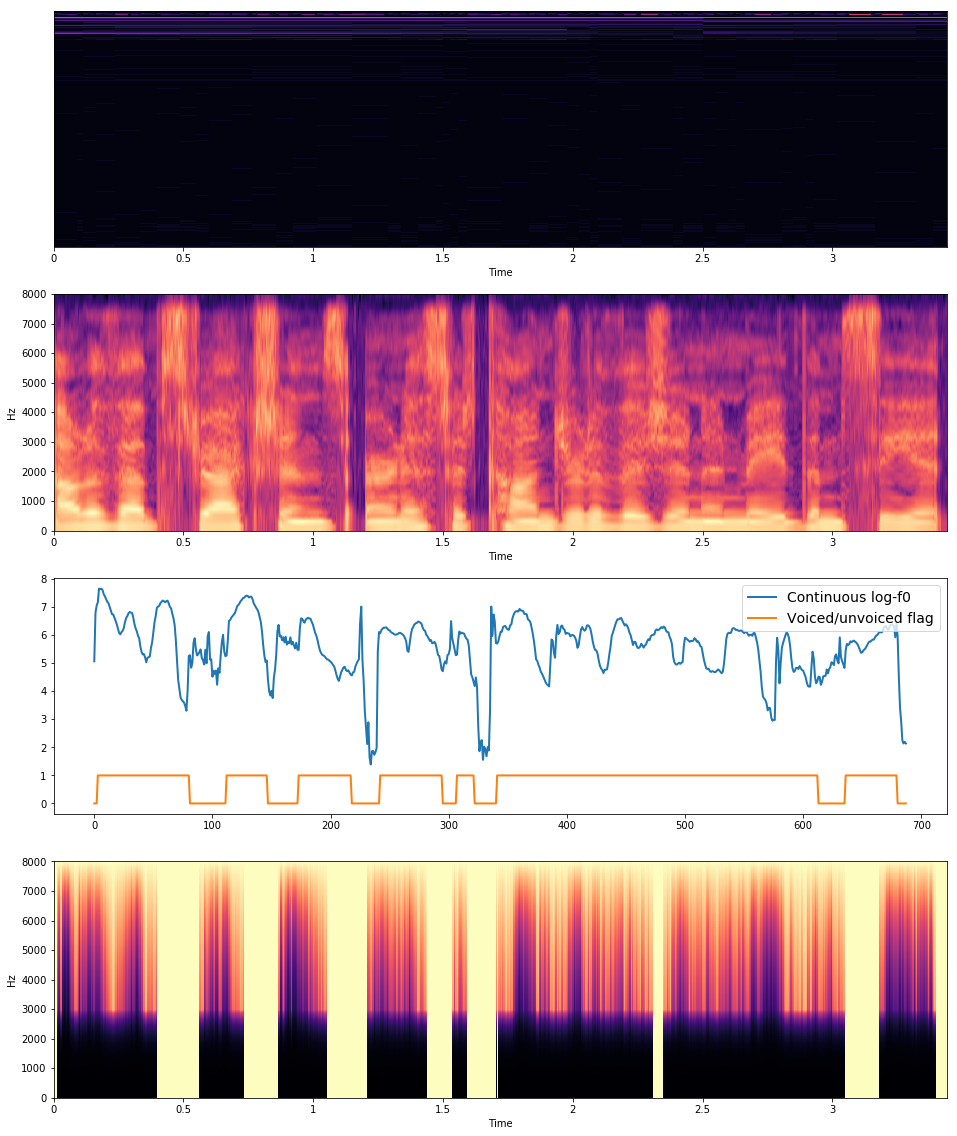As you can see the top of the images, linguistic features are not clear. This is because linguistic features have very different scale for each dimension. This will be clear after normalization.

### Statistics¶

Before training neural networks, we need to normalize data. Following Merlin’s demo script, we will apply min/max normalization for linguistic features and mean/variance normalization to duration/acoustic features. You can compute necessary statistics using nnmnkwii.preprocessing.minmax and nnmnkwii.preprocessing.meanvar. The comptuation is online, so we can use the functionality for any large dataset.

:

X_min = {}
X_max = {}
Y_mean = {}
Y_var = {}
Y_scale = {}

for typ in ["acoustic", "duration"]:
X_min[typ], X_max[typ] = minmax(X[typ]["train"], utt_lengths[typ]["train"])
Y_mean[typ], Y_var[typ] = meanvar(Y[typ]["train"], utt_lengths[typ]["train"])
Y_scale[typ] = np.sqrt(Y_var[typ])


Linguistic features should be clear with normalization as shown below.

:

idx = 0
typ = "acoustic"
x = X[typ]["train"][idx][:utt_lengths[typ]["train"][idx]]
x = minmax_scale(x, X_min[typ], X_max[typ], feature_range=(0.01, 0.99))
librosa.display.specshow(x.T, sr=fs, hop_length=hop_length, x_axis="time")
colorbar()

:

<matplotlib.colorbar.Colorbar at 0x7f77d19fd630>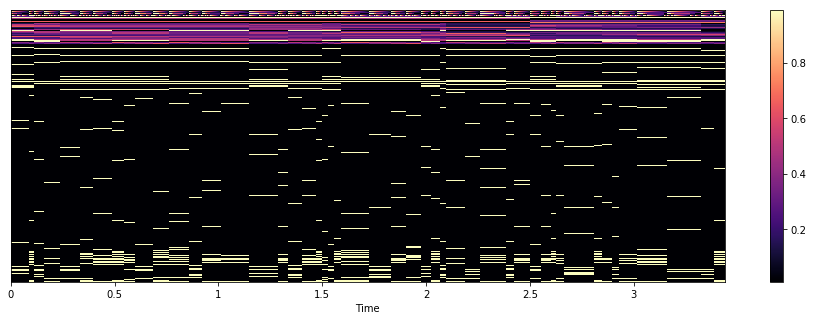### Combine datasets and normalization.¶

In this demo we use PyTorch to build DNNs. PyTorchDataset is just a glue Dataset wrapper, which combines our dataset and normalization. Note that since our dataset is zero-padded, we need its lengths.

:

from torch.utils import data as data_utils
import torch

class PyTorchDataset(torch.utils.data.Dataset):
"""Thin dataset wrapper for pytorch

This does just two things:
1. On-demand normalization
2. Returns torch.tensor instead of ndarray
"""
def __init__(self, X, Y, lengths, X_min, X_max, Y_mean, Y_scale):
self.X = X
self.Y = Y
if isinstance(lengths, list):
lengths = np.array(lengths)[:,None]
elif isinstance(lengths, np.ndarray):
lengths = lengths[:,None]
self.lengths = lengths
self.X_min = X_min
self.X_max = X_max
self.Y_mean = Y_mean
self.Y_scale = Y_scale
def __getitem__(self, idx):
x, y = self.X[idx], self.Y[idx]
x = minmax_scale(x, self.X_min, self.X_max, feature_range=(0.01, 0.99))
y = scale(y, self.Y_mean, self.Y_scale)
l = torch.from_numpy(self.lengths[idx])
x, y = torch.from_numpy(x), torch.from_numpy(y)
return x, y, l
def __len__(self):
return len(self.X)


## Model¶

We use bidirectional LSTM-based RNNs. Using PyTorch, it’s very easy to implement. To handle variable length sequences in mini-batch, we can use PackedSequence.

:

import torch
from torch import nn
from tqdm import tnrange, tqdm
import torch
import torch.nn as nn
from torch import optim

:

class MyRNN(nn.Module):
def __init__(self, D_in, H, D_out, num_layers=1, bidirectional=True):
super(MyRNN, self).__init__()
self.hidden_dim = H
self.num_layers = num_layers
self.num_direction =  2 if bidirectional else 1
self.lstm = nn.LSTM(D_in, H, num_layers, bidirectional=bidirectional, batch_first=True)
self.hidden2out = nn.Linear(self.num_direction*self.hidden_dim, D_out)

def init_hidden(self, batch_size):
h, c = (Variable(torch.zeros(self.num_layers * self.num_direction, batch_size, self.hidden_dim)),
Variable(torch.zeros(self.num_layers * self.num_direction, batch_size, self.hidden_dim)))
return h,c

def forward(self, sequence, lengths, h, c):
output, (h, c) = self.lstm(sequence, (h, c))
output = self.hidden2out(output)
return output


## Train¶

### Configurations¶

Network hyper parameters and training configurations (learning rate, weight decay, etc).

:

num_hidden_layers = 3
hidden_size = 512

batch_size = 32
n_workers = 2
pin_memory = True
nepoch = 25
lr = 0.001
weight_decay = 1e-6
use_cuda = torch.cuda.is_available()
print(use_cuda)

True


### Trainining loop¶

Our RNN predicts output feature sequence given a input feature sequence, so we need to feed our data to network in sequence-wise manner. This is pretty easy. We can just use MemoryCacheDataset that supports utterancew-wise iteration and has cache functionality to avoid file re-loading.

:

def train_rnn(model, optimizer, X, Y, X_min, X_max, Y_mean, Y_scale,
utt_lengths, cache_size=1000):
if use_cuda:
model = model.cuda()

X_train, X_test = X["train"], X["test"]
Y_train, Y_test = Y["train"], Y["test"]
train_lengths, test_lengths = utt_lengths["train"], utt_lengths["test"]

X_train_cache_dataset = MemoryCacheDataset(X_train, cache_size)
Y_train_cache_dataset = MemoryCacheDataset(Y_train, cache_size)
train_dataset = PyTorchDataset(X_train_cache_dataset, Y_train_cache_dataset, train_lengths,
X_min, X_max, Y_mean, Y_scale)
train_dataset, batch_size=batch_size, num_workers=n_workers, shuffle=True)

X_test_cache_dataset = MemoryCacheDataset(X_test, cache_size)
Y_test_cache_dataset = MemoryCacheDataset(Y_test, cache_size)
test_dataset = PyTorchDataset(X_test_cache_dataset, Y_test_cache_dataset, test_lengths,
X_min, X_max, Y_mean, Y_scale)
test_dataset, batch_size=batch_size, num_workers=n_workers, shuffle=True)

# Training loop
criterion = nn.MSELoss()
model.train()
print("Start utterance-wise training...")
loss_history = {"train": [], "test": []}
for epoch in tnrange(nepoch):
for phase in ["train", "test"]:
running_loss = 0
for x, y, lengths in dataset_loaders[phase]:
# Sort by lengths . This is needed for pytorch's PackedSequence
sorted_lengths, indices = torch.sort(lengths.view(-1), dim=0, descending=True)
sorted_lengths = sorted_lengths.long().numpy()
# Get sorted batch
x, y = x[indices], y[indices]
# Trim outputs with max length
y = y[:, :sorted_lengths]

# Init states
h, c = model.init_hidden(len(sorted_lengths))
if use_cuda:
x, y = x.cuda(), y.cuda()
if use_cuda:
h, c = h.cuda(), c.cuda()
x, y = Variable(x), Variable(y)

# Do apply model for a whole sequence at once
# no need to keep states
y_hat = model(x, sorted_lengths, h, c)
loss = criterion(y_hat, y)
if phase == "train":
loss.backward()
optimizer.step()
running_loss += loss.item()

return loss_history


### Define models¶

:

models = {}
for typ in ["duration", "acoustic"]:
models[typ] = MyRNN(X[typ]["train"].shape[-1],
hidden_size, Y[typ]["train"].shape[-1],
num_hidden_layers, bidirectional=True)
print("Model for {}\n".format(typ), models[typ])

Model for duration
MyRNN (
(lstm): LSTM(416, 512, num_layers=3, batch_first=True, bidirectional=True)
(hidden2out): Linear (1024 -> 5)
)
Model for acoustic
MyRNN (
(lstm): LSTM(425, 512, num_layers=3, batch_first=True, bidirectional=True)
(hidden2out): Linear (1024 -> 187)
)


### Training Duration model¶

:

ty = "duration"
loss_history = train_rnn(models[ty], optimizer, X[ty], Y[ty],
X_min[ty], X_max[ty], Y_mean[ty], Y_scale[ty], utt_lengths[ty])

Start utterance-wise training...



:

plot(loss_history["train"], linewidth=2, label="Train loss")
plot(loss_history["test"], linewidth=2, label="Test loss")
legend(prop={"size": 16})

:

<matplotlib.legend.Legend at 0x7f77d1950ac8>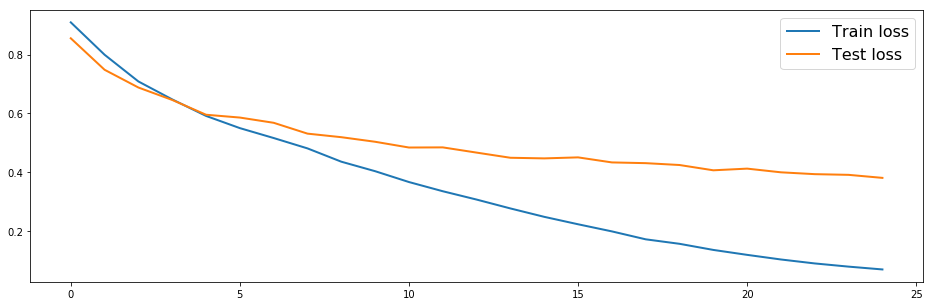### Training acoustic model¶

:

ty = "acoustic"
loss_history = train_rnn(models[ty], optimizer, X[ty], Y[ty],
X_min[ty], X_max[ty], Y_mean[ty], Y_scale[ty], utt_lengths[ty])

Start utterance-wise training...



:

plot(loss_history["train"], linewidth=2, label="Train loss")
plot(loss_history["test"], linewidth=2, label="Test loss")
legend(prop={"size": 16})

:

<matplotlib.legend.Legend at 0x7f77d18176a0>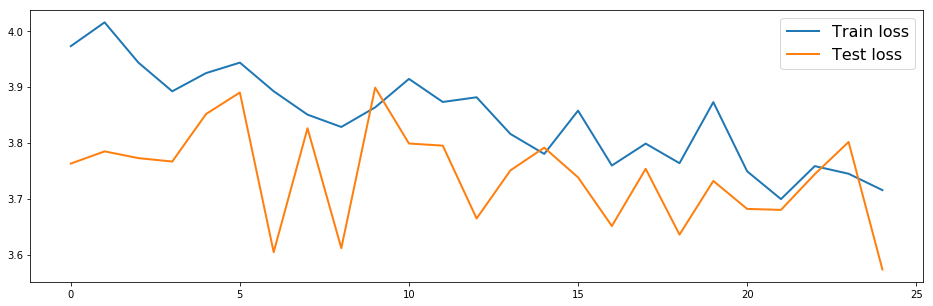## Test¶

Let’s see how our network works.

### Parameter generation utilities¶

Almost same as DNN text-to-speech synthesis. The difference is that we need to give initial hidden states explicitly.

:

binary_dict, continuous_dict = hts.load_question_set(join(DATA_ROOT, "questions-radio_dnn_416.hed"))

def gen_parameters(y_predicted):
# Number of time frames
T = y_predicted.shape

# Split acoustic features
mgc = y_predicted[:,:lf0_start_idx]
lf0 = y_predicted[:,lf0_start_idx:vuv_start_idx]
vuv = y_predicted[:,vuv_start_idx]
bap = y_predicted[:,bap_start_idx:]

# Perform MLPG
ty = "acoustic"
mgc_variances = np.tile(Y_var[ty][:lf0_start_idx], (T, 1))
mgc = paramgen.mlpg(mgc, mgc_variances, windows)
lf0_variances = np.tile(Y_var[ty][lf0_start_idx:vuv_start_idx], (T,1))
lf0 = paramgen.mlpg(lf0, lf0_variances, windows)
bap_variances = np.tile(Y_var[ty][bap_start_idx:], (T, 1))
bap = paramgen.mlpg(bap, bap_variances, windows)

return mgc, lf0, vuv, bap

def gen_waveform(y_predicted, do_postfilter=False):
y_predicted = trim_zeros_frames(y_predicted)

# Generate parameters and split streams
mgc, lf0, vuv, bap = gen_parameters(y_predicted)

if do_postfilter:
mgc = merlin_post_filter(mgc, alpha)

spectrogram = pysptk.mc2sp(mgc, fftlen=fftlen, alpha=alpha)
aperiodicity = pyworld.decode_aperiodicity(bap.astype(np.float64), fs, fftlen)
f0 = lf0.copy()
f0[vuv < 0.5] = 0
f0[np.nonzero(f0)] = np.exp(f0[np.nonzero(f0)])

generated_waveform = pyworld.synthesize(f0.flatten().astype(np.float64),
spectrogram.astype(np.float64),
aperiodicity.astype(np.float64),
fs, frame_period)
return generated_waveform

def gen_duration(label_path, duration_model):
# Linguistic features for duration
duration_linguistic_features = fe.linguistic_features(hts_labels,
binary_dict, continuous_dict,
subphone_features=None).astype(np.float32)

# Apply normalization
ty = "duration"
duration_linguistic_features = minmax_scale(duration_linguistic_features,
X_min[ty], X_max[ty], feature_range=(0.01, 0.99))

# Apply models
duration_model = duration_model.cpu()
duration_model.eval()

#  Apply model
x = Variable(torch.from_numpy(duration_linguistic_features)).float()
try:
duration_predicted = duration_model(x).data.numpy()
except:
h, c = duration_model.init_hidden(batch_size=1)
xl = len(x)
x = x.view(1, -1, x.size(-1))
duration_predicted = duration_model(x, [xl], h, c).data.numpy()
duration_predicted = duration_predicted.reshape(-1, duration_predicted.shape[-1])

# Apply denormalization
duration_predicted = duration_predicted * Y_scale[ty] + Y_mean[ty]
duration_predicted = np.round(duration_predicted)

# Set minimum state duration to 1
duration_predicted[duration_predicted <= 0] = 1
hts_labels.set_durations(duration_predicted)

return hts_labels

def test_one_utt(label_path, duration_model, acoustic_model, post_filter=True):
# Predict durations
duration_modified_hts_labels = gen_duration(label_path, duration_model)

# Linguistic features
linguistic_features = fe.linguistic_features(duration_modified_hts_labels,
binary_dict, continuous_dict,
subphone_features="full")
# Trim silences
indices = duration_modified_hts_labels.silence_frame_indices()
linguistic_features = np.delete(linguistic_features, indices, axis=0)

# Apply normalization
ty = "acoustic"
linguistic_features = minmax_scale(linguistic_features,
X_min[ty], X_max[ty], feature_range=(0.01, 0.99))

# Predict acoustic features
acoustic_model = acoustic_model.cpu()
acoustic_model.eval()
x = Variable(torch.from_numpy(linguistic_features)).float()
try:
acoustic_predicted = acoustic_model(x).data.numpy()
except:
h, c = acoustic_model.init_hidden(batch_size=1)
xl = len(x)
x = x.view(1, -1, x.size(-1))
acoustic_predicted = acoustic_model(x, [xl], h, c).data.numpy()
acoustic_predicted = acoustic_predicted.reshape(-1, acoustic_predicted.shape[-1])

# Apply denormalization
acoustic_predicted = acoustic_predicted * Y_scale[ty] + Y_mean[ty]

return gen_waveform(acoustic_predicted, post_filter)


### Listen generated audio¶

Generated audio samples with Merlin’s slt_full_demo are attached. You can compare them below.

:

test_label_paths = sorted(glob(join(DATA_ROOT, "label_state_align/", "*.lab")))[::-1][:5][::-1]
merlin_generated_wav_files = sorted(glob(join("./generated/merlin_slt_arctic_full/*.wav")))
ffn_generated_wav_files = sorted(glob(join("./generated/01-tts/*.wav")))

# Save generated wav files for later comparizon
save_dir = join("./generated/02-tts")
if not exists(save_dir):
os.makedirs(save_dir)

for label_path, wav_path1, wav_path2 in zip(test_label_paths,
ffn_generated_wav_files,
merlin_generated_wav_files):
print("MyNet (from 01-tts demo notebook)")
IPython.display.display(Audio(waveform, rate=fs))

print("MyRNN")
waveform = test_one_utt(label_path, models["duration"], models["acoustic"])
wavfile.write(join(save_dir, basename(wav_path1)), rate=fs, data=waveform)
IPython.display.display(Audio(waveform, rate=fs))

print("Merlin (slt_arctic_full, generated with default configurations)")
IPython.display.display(Audio(waveform, rate=fs))

MyNet (from 01-tts demo notebook)

MyRNN

Merlin (slt_arctic_full, generated with default configurations)

MyNet (from 01-tts demo notebook)

MyRNN

Merlin (slt_arctic_full, generated with default configurations)

MyNet (from 01-tts demo notebook)

MyRNN

Merlin (slt_arctic_full, generated with default configurations)

MyNet (from 01-tts demo notebook)

MyRNN